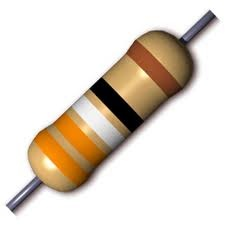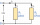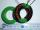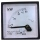# Resistance

A resistor having an electrical resistance of 1.5 k ohms passes an electrical current of 0.1 A. Calculate what voltage is between the terminals of the resistor.

U =  150 V

### Step-by-step explanation:Did you find an error or inaccuracy? Feel free to write us. Thank you!## Related math problems and questions:

• ResistorResistor 1 with a resistance of 100 Ohms and resistor 2 with a resistance of 400 Ohms are connected side by side in the circuit. There is a voltage of 80V between the resistor terminals. a) Draw a circuit diagram and write the entered quantities in it b)
• Circuit voltageCalculate the closed-circuit voltage. Calculate the power on the resistors. R1 = 30 ohms, R2 = 10 ohms. I = 0.1A. U =?. The resistors are connected in series, one after the other.
• Resistance of the resistorThe resistor terminals have a voltage of 20 V and a current of 5 mA is passed through. What is the resistance of the resistor?
• Resistance - ohmsIf the resistance of the first resistor is 300 ohms and the resistance of the second resistor is 100 ohms. What is the resulting resistance?
• Coils of transformerThe primary coil of the transformer has 400 turns, a current of 1.5 A passes through it and is connected to a voltage of 220 V. For the secondary coil, find the voltage, current, and a number of turns if the transformation ratio k = 0.1.
• Closed circuitIn a closed circuit, there is a voltage source with U1 = 12 V and with an internal resistance R1 = 0.2 Ω. The external resistance is R2 = 19.8 Ω. Determine the electric current and terminal voltage.
• Current in the conductorCalculate the current in the conductor (in mA) if it is connected to a 4.5 V voltage source and its resistance is 20 (ohm).
• Coil as a girlThe electrical resistance of the copper wire coil is 2.0 ohms. What current runs through the coil when the voltage between the terminals is 3.0 V?
• The resistanceWhat is the resistance of an aluminum wire 0.2 km long and 10 mm in diameter?
• Direction indicatorAs that which the electrical voltage is connected to the lamp direction indicator 7400 mOhm resistance when current of 1.6 A passes through it?
• Kirchhoff's lawTwo resistors with 100 Ω and 300 Ω resistors are connected in series. A current of 1.8 A passes through the first 100Ω resistor. What current flows through the second resistor?
• Mains voltageHow much electric current flows through the appliance with a resistance of 40kΩ, which is connected to the mains voltage (230 V)?
• The bulbsThe bulbs are connected serially two bulbs and one resistor. Each bulb has a resistivity of 100 ohms and resistor of 60 ohms The voltage source in the circuit is 24 volts. Do you know what voltage to measure on individual appliances?
• Serial connectionIf the voltage on the first resistor is 6 V and the resistance ratio of the first and second resistors is 1: 3, what will be the voltage across the second resistor if they are connected in series?
• CookerA current of 2A passes through the immersion cooker at a voltage of 230V. What work do the electric field forces in 2 minutes?
• Electric input powerSolve problems related to electric power: a) U = 120 V, I = 0.5 A, P =? b) P = 200 W, U = 230 V, I =? c) I = 5 A, P = 2200W, U =?
• ResistanceDetermine the resistance of the bulb with the current 200 mA and is in a regular lamp (230V).